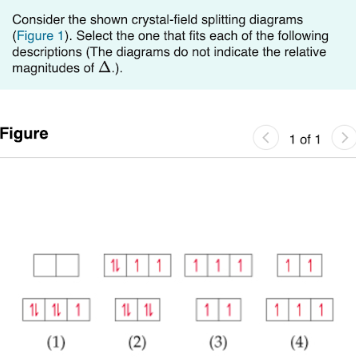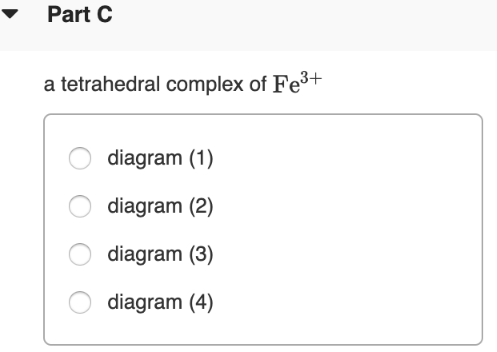# Consider the shown crystal-field splitting diagrams (Figure 1.) Select the one that fits each of the following descriptions. (The diagrams do not indicate the relative magnitudes of Δ.). A tetrahedral complex Fe^3+ a) diagram (1) b) diagram (2) c) diagram (3) d) diagram (4)